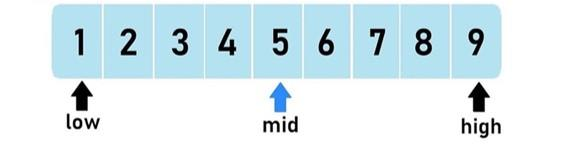Loading, please wait...# The first basic algorithm is binary search

May 05, 2020 ML, algorithm, binary search, 3215 Views

Hi! Today I wanna start writing some short tips for different algorithms. The first basic algorithm is a binary search🙃

It helps you to find the element in a sorted array.
This algorithm is one of the simplest. It can be understood by looking at the next picture▶️Swipe▶️ Imagine we are looking for a number X in sorted array A.1. We put left (L) and right (R) “arrows” on the first and last elements of the sorted array.
2. Then the middle (M) “arrow” is on the center of array: M = (L + R) / 2
3. If the number in the place M is bigger then X, then we move arrow R to M. Else the arrow L to M (if a[M] > X then R = M, else L = M)
4. Continue until L + 1 != R.
5. The answer is on the element L

Easy? Yes! And the complexity of the binary search is O(log(n)), where n = length of an array. So, if n = 10^6, then you do ~20 operations instead of 10^6.

Here you can find problems on this algorithm: https://leetcode.com/tag/binary-search/
Try to solve it😛